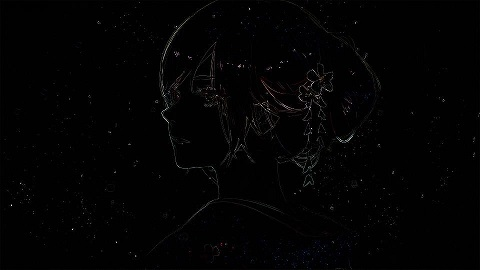锐化滤波

${\nabla }^{2}f=\frac{{\partial }^{2}f}{\partial {x}^{2}}+\frac{{\partial }^{f}}{\partial {y}^{2}}$

$\frac{{\partial }^{2}f}{\partial {x}^{2}}=f\left(x+1,y\right)+f\left(x-1,y\right)-2f\left(x,y\right)$

$\frac{{\partial }^{2}f}{\partial {y}^{2}}=f\left(x,y+1\right)+f\left(x,y-1\right)-2f\left(x,y\right)$

${\nabla }^{2}f=f\left(x+1,y\right)+f\left(x-1,y\right)+f\left(x,y+1\right)+f\left(x,y-1\right)-4f\left(x,y\right)$

$\left[\begin{array}{ccc}0& 1& 0\\ 1& -4& 1\\ 0& 1& 0\end{array}\right]$

$\left[\begin{array}{ccc}0& 1& 0\\ 1& -4& 1\\ 0& 1& 0\end{array}\right]\left[\begin{array}{ccc}0& -1& 0\\ -1& 4& -1\\ 0& -1& 0\end{array}\right]\left[\begin{array}{ccc}-1& -1& -1\\ -1& 8& -1\\ -1& -1& -1\end{array}\right]\left[\begin{array}{ccc}1& 1& 1\\ 1& -8& 1\\ 1& 1& 1\end{array}\right]$

$g\left(x,y\right)=\left\{\begin{array}{c}f\left(x,y\right)-{\nabla }^{2}f\left(x,y\right)\phantom{\rule{1ex}{0ex}}if\phantom{\rule{1ex}{0ex}}\text{拉普拉斯算子中心系数为负}\\ f\left(x,y\right)+{\nabla }^{2}f\left(x,y\right)\phantom{\rule{1ex}{0ex}}if\phantom{\rule{1ex}{0ex}}\text{拉普拉斯算子中心系数为正}\end{array}$

代码实现

$g\left(x,y\right)=f\left(x,y\right)-{\nabla }^{2}f\left(x,y\right)=5f\left(x,y\right)-\left[f\left(x+1,y\right)+f\left(x-1,y\right)+f\left(x,y+1\right)+f\left(x,y-1\right)\right]$

``````import numpy as np
import PIL.Image
import scipy.misc
import scipy.signal

def convert_2d(r):
# 滤波掩模
window = np.array([
[0, -1, 0],
[-1, 5, -1],
[0, -1, 0]
])
s = scipy.signal.convolve2d(r, window, mode='same', boundary='symm')
# 像素值如果大于 255 则取 255, 小于 0 则取 0
for i in range(s.shape):
for j in range(s.shape):
s[i][j] = min(max(0, s[i][j]), 255)
s = s.astype(np.uint8)
return s

def convert_3d(r):
s_dsplit = []
for d in range(r.shape):
rr = r[:, :, d]
ss = convert_2d(rr)
s_dsplit.append(ss)
s = np.dstack(s_dsplit)
return s

im = PIL.Image.open('/img/jp.jpg')
im_mat = scipy.misc.fromimage(im)
im_converted_mat = convert_3d(im_mat)
im_converted = PIL.Image.fromarray(im_converted_mat)
im_converted.show()
``````

效果展示